# 超临界分枝过程的大偏差速率Large Deviation Rates for Supercritical Branching Process

DOI: 10.12677/PM.2021.114076, PDF, HTML, XML, 下载: 10  浏览: 41

Abstract: Suppose {Y(t);t ≥0} is the continuous time supercritical branching process with offspring rates {bk;k≥0} and immigration rates {aj;j≥0}. Let b0=0, 0 < bk≠1(k≥1),1 < m=Σk=0 kbk < ∞, 0 < a=Σj=0 jbj < ∞ and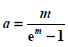. Firstly, we suppose that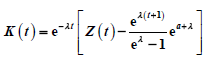is a sub-martingale and converges to a random variable K. Then we study the decay rates of P(|K(t)-K| > ε) as  t→∞ for α > 0, ε > 0 under various moment conditions on {ak;k≥0} and {bk;k≥0}.

1. 引言

$Z\left(t\right)=\underset{i=1}{\overset{Z\left(t-s\right)}{\sum }}{Z}_{t-s}^{i}\left(s\right)+{Y}_{t-s}\left(s\right),\text{\hspace{0.17em}}t>0,\text{\hspace{0.17em}}s\ge 0,\text{\hspace{0.17em}}Z\left(0\right)=1,$ (1.1)

$W\left(t\right):=\frac{X\left(t\right)}{C\left(t\right)}\stackrel{\text{a}\text{.s}\text{.}}{\to }W,\text{\hspace{0.17em}}$$t\to \infty$ (1.2)

$V\left(t\right):=\frac{Z\left(t\right)}{C\left(t\right)}\to V\text{\hspace{0.17em}}\text{a}.\text{s}.,$$t\to \infty$ (1.3)

$\begin{array}{l}C\left(0\right)=1,\\ C\left(t\right)\to \infty ,\text{}\frac{C\left(t+s\right)}{C\left(t\right)}\to m\left(s\right),\text{}t\to \infty ,\\ {c}_{1}\left(\frac{{\text{e}}^{\lambda }}{1+\epsilon }\right)\le C\left(t\right)\le {c}_{2}{\text{e}}^{\lambda t},\text{}t\ge N\left(\epsilon \right).\end{array}$ (1.4)

${q}_{ij}:=\left\{\begin{array}{ll}i{b}_{j-i+1}+{a}_{j-i}\hfill & \text{if}\text{\hspace{0.17em}}i\ge 0,j\ge i,\hfill \\ i{b}_{0}\hfill & \text{if}\text{\hspace{0.17em}}i\ge 0,j=i-1,\hfill \\ 0\hfill & \text{otherwise}\text{.}\hfill \end{array}$

${b}_{j}\ge 0\left(j\ne 1\right)$$0<-{b}_{1}=\underset{j\ne 1}{\sum }{b}_{j}<\infty$

${a}_{j}\ge 0\left(j\ne 0\right)$$0<-{a}_{0}=\underset{j\ne 0}{\sum }{a}_{j}<\infty$

$G\left(s,t\right)={G}_{1}\left(s,t\right)=F\left(s,t\right)H\left(s,t\right),\text{\hspace{0.17em}}t>0,\text{\hspace{0.17em}}s\in \left[0,1\right],$ (1.5)

$R\left(s,t\right):=\frac{H\left(s,t\right)}{{\text{e}}^{{a}_{0}t}}$$Q\left(s,t\right):=\frac{F\left(s,t\right)}{{\text{e}}^{{b}_{1}t}}$

$t\to \infty$ 时，

$\varrho \left(s,t\right):=\frac{G\left(s,t\right)}{{\text{e}}^{\left({a}_{0}+{b}_{1}\right)t}}=R\left(s,t\right)Q\left(s,t\right)↗R\left(s\right)Q\left(s\right)=:\varrho \left(s\right),$ (1.6)

$G\left(s\right)R\left(F\left(s\right)\right)={\text{e}}^{{a}_{0}}R\left(s\right)$$0\le s<1$$R\left(0\right)=1$$R\left(1\right)=\infty$

$Q\left(F\left(s\right)\right)={\text{e}}^{{b}_{1}}Q\left(s\right)$$0\le s<1$$Q\left(0\right)=0$$Q\left(1\right)=\infty$

$K\left(t\right)={\text{e}}^{-\lambda t}\left[Z\left(t\right)-\frac{{\text{e}}^{\lambda \left(t+1\right)}-1}{{\text{e}}^{\lambda }-1}{\text{e}}^{a+\lambda }\right],$ (1.7)

$P\left(|K\left(t\right)-K|>\epsilon \right),\text{\hspace{0.17em}}t\to \infty .$

$\underset{t\to \infty }{\mathrm{lim}}{\text{e}}^{-\left({b}_{1}+{a}_{0}\right)t}P\left(|\frac{Z\left(t+v\right)}{Z\left(t\right)}-{\text{e}}^{\lambda v}|>\epsilon |Z\left(0\right)=1\right)=\underset{l=1}{\overset{\infty }{\sum }}\varphi \left(v,l,\epsilon \right){\rho }_{l}<\infty ,$ (1.8)

$\varphi \left(v,l,\epsilon \right)=P\left(|\stackrel{¯}{{X}_{l}}\left(v\right)+\frac{Y\left(v\right)}{l}-{\text{e}}^{\lambda v}|>\epsilon \right),$

$\stackrel{¯}{{X}_{l}}\left(v\right)={\sum }_{j=1}^{l}\frac{{X}_{j}\left(v\right)}{l}$$\left\{{\rho }_{l}\right\}$ 是引理2.2中定义的。

${C}_{1}={\mathrm{sup}}_{t\ge 0}E\left[{\text{e}}^{{\theta }_{1}K\left(t\right)}\right]<\infty .$

$P\left(|K\left(t\right)-K|>\epsilon \right)\le {C}_{2}{\text{e}}^{-\xi {\epsilon }^{2/3}{\text{e}}^{\lambda t/3}}.$

2. 预备知识

$\underset{t\to \infty }{\mathrm{lim}}{\text{e}}^{-bt}{\int }_{0}^{t}{\text{e}}^{\left(\eta +b\right)v}{p}_{jk}\left(v\right)\text{d}v={b}^{-1}{\varphi }_{jk}$

$\left({b}_{1}+{a}_{0}\right)\varrho \left(w\right)=B\left(w\right){\varrho }^{\prime }\left(w\right)+A\left(w\right)\varrho \left(w\right),\text{\hspace{0.17em}}0\le w\le 1,$ (2.1)

${{p}^{\prime }}_{1k}\left(t\right)=\underset{i=1}{\overset{k}{\sum }}{p}_{1i}\left(t\right)\left(i{b}_{k-i+1}+{a}_{k-i}\right),\text{\hspace{0.17em}}k\ge 1.$ (2.2)

$k=1$ 时，

${{p}^{\prime }}_{11}\left(t\right)={p}_{11}\left(t\right)\left({b}_{1}+{a}_{0}\right),$

${p}_{11}\left(t\right)={\text{e}}^{\left({b}_{1}+{a}_{0}\right)t},$

${\rho }_{1}=\underset{t\to \infty }{\mathrm{lim}}{\text{e}}^{-\left({b}_{1}+{a}_{0}\right)t}{p}_{11}\left(t\right)=1.$

$k=2$ 时，

${{p}^{\prime }}_{12}\left(t\right)={p}_{11}\left(t\right)\left({b}_{2}+{a}_{1}\right)+{p}_{12}\left(t\right)\left(2{b}_{1}+{a}_{0}\right),$

${\text{e}}^{-\left(2{b}_{1}+{a}_{0}\right)t}{p}_{12}\left(t\right)=\left({b}_{2}+{a}_{1}\right){\int }_{0}^{t}{p}_{11}\left(v\right){\text{e}}^{-\left(2{b}_{1}+{a}_{0}\right)v}\text{d}v,$

${\rho }_{2}=\underset{t\to \infty }{\mathrm{lim}}{\text{e}}^{-\left({b}_{1}+{a}_{0}\right)t}{p}_{12}\left(t\right)=\left({b}_{2}+{a}_{1}\right){\int }_{0}^{t}{p}_{11}\left(v\right){\text{e}}^{-\left(2{b}_{1}+{a}_{0}\right)v}\text{d}v=-\frac{{\rho }_{1}\left({b}_{2}+{a}_{1}\right)}{{b}_{1}}\le {\rho }_{1}.$

$k=k$ 时，

${{p}^{\prime }}_{1k}\left(t\right)=\underset{i=1}{\overset{k-1}{\sum }}{p}_{1i}\left(t\right)\left(i{b}_{k-i+1}+{a}_{k-i}\right)+{p}_{1k}\left(t\right)\left(k{b}_{1}+{a}_{0}\right),$

${\text{e}}^{-\left(k{b}_{1}+{a}_{0}\right)t}{p}_{1k}\left(t\right)=\underset{i=1}{\overset{k-1}{\sum }}\left(i{b}_{k-i+1}+{a}_{k-i}\right){\int }_{0}^{t}{p}_{1i}\left(v\right){\text{e}}^{-\left(k{b}_{1}+{a}_{0}\right)v}\text{d}v,$

${\rho }_{k}=\underset{t\to \infty }{\mathrm{lim}}{\text{e}}^{-\left({b}_{1}+{a}_{0}\right)t}{p}_{1k}\left(t\right)=-\frac{1}{\left(k-1\right){b}_{1}}\underset{i=1}{\overset{k-1}{\sum }}\left(i{b}_{k-i+1}+{a}_{k-i}\right){\rho }_{i}\le {\rho }_{1}.$

$\underset{k=0}{\overset{\infty }{\sum }}{\text{e}}^{-\left({b}_{1}+{a}_{0}\right)t}{{p}^{\prime }}_{jk}\left(t\right){s}^{k}=B\left(v\right)\underset{k=1}{\overset{\infty }{\sum }}{\text{e}}^{-\left({b}_{1}+{a}_{0}\right)t}{p}_{jk}\left(t\right)k{v}^{k-1}+A\left(v\right)\underset{k=0}{\overset{\infty }{\sum }}{\text{e}}^{-\left({b}_{1}+{a}_{0}\right)t}{p}_{jk}\left(t\right){v}^{k},$

${\left\{\underset{k=0}{\overset{\infty }{\sum }}{\text{e}}^{-\left({b}_{1}+{a}_{0}\right)t}{p}_{jk}\left(t\right){v}^{k}\right\}}^{\prime }{}_{t}+\left({b}_{1}+{a}_{0}\right)\underset{k=0}{\overset{\infty }{\sum }}{\text{e}}^{-\left({b}_{1}+{a}_{0}\right)t}{p}_{jk}\left(t\right){v}^{k}=B\left(v\right)\underset{k=1}{\overset{\infty }{\sum }}{\text{e}}^{-\left({b}_{1}+{a}_{0}\right)t}{p}_{jk}\left(t\right)k{v}^{k-1}+A\left(v\right)\underset{k=0}{\overset{\infty }{\sum }}{\text{e}}^{-\left({b}_{1}+{a}_{0}\right)t}{p}_{jk}\left(t\right){v}^{k}.$

$t\to \infty$

$\left({b}_{1}+{a}_{0}\right)\varrho \left(v\right)=B\left(v\right){\varrho }^{\prime }\left(v\right)+A\left(v\right)\varrho \left(v\right).$

$Z\left(t+v\right)={\sum }_{i=1}^{Z\left(t\right)}{\xi }_{t,i}\left(v\right)+Y\left(v\right),$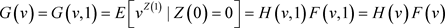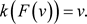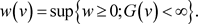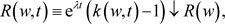(2.3)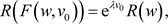(2.4)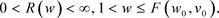(2.5)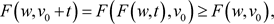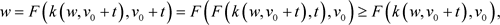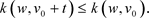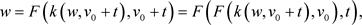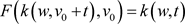a.s.,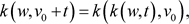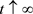时，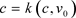。因此我们可知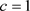也就是当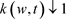。现在，对于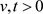时，存在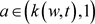使得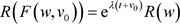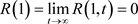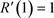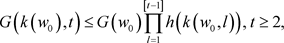(2.6)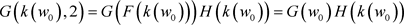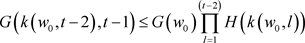3. 定理证明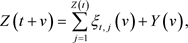(3.1)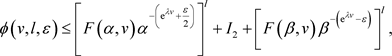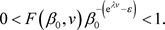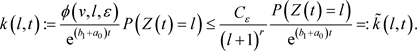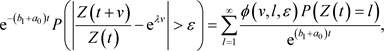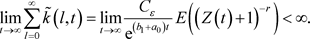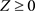时，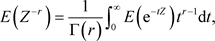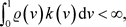(3.2)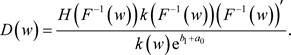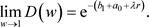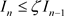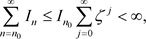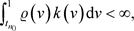(3.3)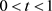时，有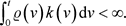(3.4)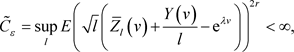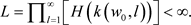(3.5)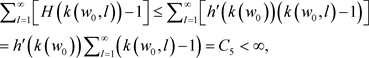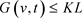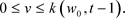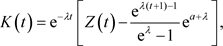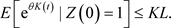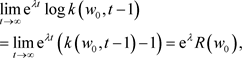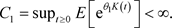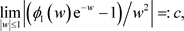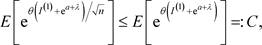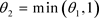，则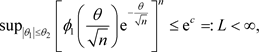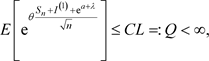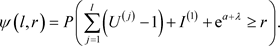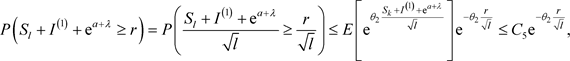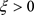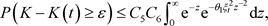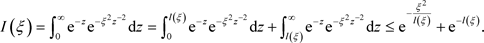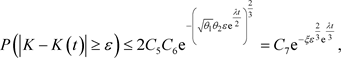Athreya, K.B. and Ney, P.E. (1972) Branching Processes. Springer-Verlag, Berlin. https://doi.org/10.1007/978-3-642-65371-1  Athreya, K.B. (1994) Large Deviation Rates for Branching Process—I. Single Type Case. The Annals of Applied Probability, 4, 779-790. https://doi.org/10.1214/aoap/1177004971  Seneta, E. (1970) On the Supercritical Galton-Watson Process with Immigration. Mathematical Biosciences, 7, 9-14. https://doi.org/10.1016/0025-5564(70)90038-6  Liu, J. and Zhang, M. (2016) Large Deviation for Supercritical Branching Processes. Acta Mathematica Sinica, English Series, 32, 893-900. https://doi.org/10.1007/s10114-016-5437-z  Ney, P.E. and Vidyashankar, A.N. (2003) Harmonic Moments and Large Deviation Rates for Supercritical Branching Processes. The Annals of Applied Probability, 13, 475-489. https://doi.org/10.1214/aoap/1050689589